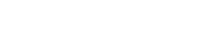College GPA CalculatorFinal grade calculatorGrade calculator

# COLLEGE GPA

Use the Ultra Fast and Free College GPA Calculator to calculate your
current Semester's GPA and Cumulative GPA.

Semester 1
0.00

A

A  =  4.00

A-  =  3.67

B

B+  =  3.33

B  =  3.00

B-  =  2.67

C

C+  =  2.33

C  =  2.00

C-  =  1.67

D

D+  =  1.33

D  =  1.00

D-  =  0.67

F

F  =  0

In today's competitive academic and work environments, it's very important to monitor your college GPA. There are different ways around the world in which learning institutions track a students academic performance. College GPA Calculator only uses the letter and numerical systems, which is the way most Colleges and Universities in the United States grade students.